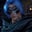Related Tags

javascript
array
communitycreator

# How to get the frequency of smallest array value in JavaScriptGutha Vamsi Krishna

### Problem

Given an array of n elements, find the frequency of the smallest value in the array.

### Example

Input: [2,2,5,5,7,9,2]

Output: 3

#### Explanation

2 is the smallest element in the given array and it appears 3 times.

### Solution

We can solve this problem with two approaches.

1. Using two loops
2. Using one loop

#### Using two loops

• Initialize the array arr.

• Initialize the frequency freq with 0.

• Initialize the minimum with the first element in the array.

• Use the first loop to find out the minimum element in the array.

• Loop through the entire array, and check if the current element is less than the value in minimum.
• If it is, then assign the current element to variable minimum.
• Now, the minimum variable contains a minimum element in the array after traversing the entire array.

• Use the second loop to find out occurrences of minimum element in the array.

• Check if the current element is equal to a minimum if it is then increment the freq by 1.
• Print the freq variable as it contains the frequency of minimum element in the array, after executing the second loop.

#### Code

//initialize array
let arr = [2,2,5,5,7,9,2]

//initialize freq and minimum
let freq = 0;
let minimum = arr;

//find the minimum element in the array
for(let i = 1 ;i < arr.length; i++){
if(arr[i] < minimum){
minimum = arr[i];
}
}

//find the frequency of minimum element resulted from above loop
for(let i = 0 ; i < arr.length ; i++){
if(arr[i] == minimum){
freq += 1;
}
}

//print the frequency of minimum element in the array
console.log(freq)

#### Using one loop

• Initialize the array arr.

• Initialize the frequency freq with 1.

• Initialize the minimum with the first element in the array.

• Loop through the array index 1 as we already assigned the first element to a minimum

• If the current element is smaller than the minimum, assign the current element to the variable minimum, and set freq to 1.
• Otherwise, if the current element is equal to a minimum then increment the freq by 1.
• Now print out the freq.

#### Code

//initialize array
let arr = [2, 2, 5, 5, 7, 9, 2]

//initialize freq and minimum
let freq = 1;
let minimum = arr;

//find the minimum element in the array
for(let i = 1; i < arr.length ; i++){
if(arr[i] < minimum){
minimum = arr[i];
freq = 1;
}else if(arr[i] == minimum){
freq += 1;
}
}

console.log(freq)

RELATED TAGS

javascript
array
communitycreator

CONTRIBUTORGutha Vamsi Krishna
RELATED COURSES

View all Courses

Keep Exploring

Learn in-demand tech skills in half the time